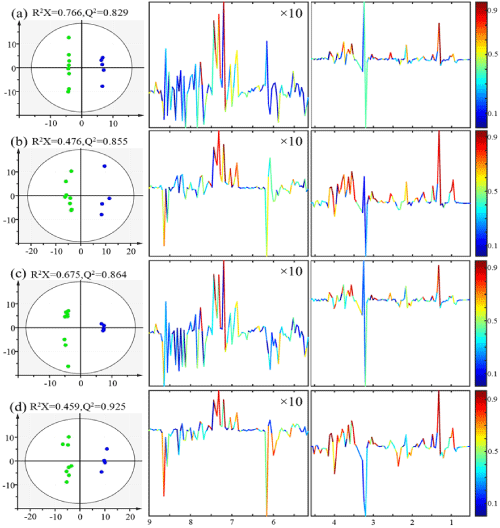Figure 3: OPLS scores (left) and coefficients-coded loading plot (right) of the model discriminating the control (green dots) and the radiation (blue dots) groups. (a) Data derived from binning results of control and 3 Gy and normalization to unit weight, (b) Data derived from binning results of control and 7.8 Gy and normalization to unit weight, (c) Data derived from binning results of control and 3 Gy and normalization to constant sum, (d) Data derived from binning results of control and 7.8 Gy and normalization to constant sum. CV-ANOVA results gave p values of 0.095, 0.046, 0.02 and 0.005 for models (a), (b), (c) and (d), respectively.